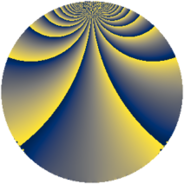# Properties

 Label 441.2.wLevel $441$ Weight $2$ Character orbit 441.w Rep. character $\chi_{441}(62,\cdot)$ Character field $\Q(\zeta_{14})$ Dimension $120$ Newform subspaces $1$ Sturm bound $112$ Trace bound $0$

# Related objects

## Defining parameters

 Level: $$N$$ $$=$$ $$441 = 3^{2} \cdot 7^{2}$$ Weight: $$k$$ $$=$$ $$2$$ Character orbit: $$[\chi]$$ $$=$$ 441.w (of order $$14$$ and degree $$6$$) Character conductor: $$\operatorname{cond}(\chi)$$ $$=$$ $$147$$ Character field: $$\Q(\zeta_{14})$$ Newform subspaces: $$1$$ Sturm bound: $$112$$ Trace bound: $$0$$

## Dimensions

The following table gives the dimensions of various subspaces of $$M_{2}(441, [\chi])$$.

Total New Old
Modular forms 360 120 240
Cusp forms 312 120 192
Eisenstein series 48 0 48

## Trace form

 $$120 q + 24 q^{4} + O(q^{10})$$ $$120 q + 24 q^{4} - 32 q^{16} - 44 q^{22} - 4 q^{25} - 56 q^{28} + 112 q^{34} - 76 q^{37} + 28 q^{40} + 8 q^{43} - 40 q^{46} - 84 q^{49} - 140 q^{52} + 12 q^{58} - 84 q^{61} + 24 q^{64} + 16 q^{67} + 112 q^{70} - 84 q^{76} - 24 q^{79} + 140 q^{82} - 96 q^{85} - 24 q^{88} - 112 q^{91} - 112 q^{94} + O(q^{100})$$

## Decomposition of $$S_{2}^{\mathrm{new}}(441, [\chi])$$ into newform subspaces

Label Dim. $$A$$ Field CM Traces $q$-expansion
$$a_2$$ $$a_3$$ $$a_5$$ $$a_7$$
441.2.w.a $$120$$ $$3.521$$ None $$0$$ $$0$$ $$0$$ $$0$$

## Decomposition of $$S_{2}^{\mathrm{old}}(441, [\chi])$$ into lower level spaces

$$S_{2}^{\mathrm{old}}(441, [\chi]) \cong$$ $$S_{2}^{\mathrm{new}}(147, [\chi])$$$$^{\oplus 2}$$